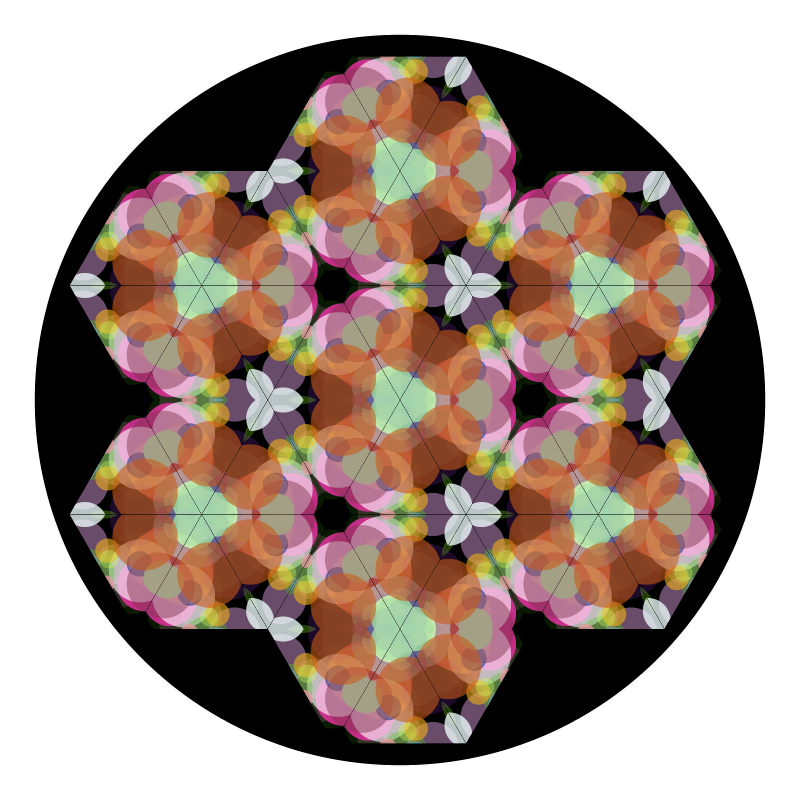A three mirror kaleidescope with random confetti.Author: Jeffrey Rosenbluth
> import Diagrams.Backend.SVG.CmdLine
> {-# LANGUAGE NoMonomorphismRestriction #-}
> import           Data.Colour.Palette.ColorSet
> import           Data.List                     (zipWith, zipWith3)
> import           Diagrams.Prelude
> import           System.Random

A helper function like iterate but also takes the list index as a parameter.

> iterateIdx :: (Int -> a -> a) -> a -> [a]
> iterateIdx f t = go f t 0
>   where
>     go f t i = let t' = f i t
>                in  t' : go f t' (i + 1)

Take any Diagram and cut out an equilateral triangle of side 1 from the center. This is the triangle inside of the three mirrors that make up a kaleidescope. The image is created by first repeatedly refecting this triangle and assembling the resulting triagles into a hexagon. Then the image plane is tiled with this hexagon.

> kaleidoscope :: Diagram B -> Diagram B
> kaleidoscope d = appends hex hexs
>   where
>     hexs   = zip dirs (replicate 6 hex)
>     dirs   = iterate (rotateBy (1/6)) (rotateBy (1/12) unitX)
>     hex    = mconcat . take 6 iterateIdx next tri > tri = alignBR cutTriangle d
>     next i = reflectAbout (0 ^& 0) (rotateBy (- fromIntegral i / 6) xDir)
> cutTriangle :: Diagram B -> Diagram B
> cutTriangle = clipped (triangle 1) # lw none

We pass as arguments the number of pieces of confetti n and a random seed r. Between 10 and 100 pieces seem to work nicely.

> confettiScope :: Int -> Int -> Diagram B
> confettiScope n r
>   = kaleidoscope (mkConfetti n (mkStdGen r))
>   # centerXY <> (circle 2.75 # fc black)
>   # pad 1.1

To create an image for use in the kadeidescope we generate a bunch of disks with random location, size, color, and opacity. This is the confetti used as the image. Of course using circles is arbitrary, any shapes and sizes will do.

> sizeValue :: (RandomGen g) => Rand g Double
> sizeValue = getRandomR (0.05, 0.25)
> coordValue :: (RandomGen g) => Rand g Double
> coordValue = getRandomR (-0.5, 0.5)

We use monadRandom to hide the plumbing of the many random numbers we need. The colors are choosen from the 330+ webColors defined in the package Data.Colour.Palette.ColorSet.

> confetti :: Int -> Rand StdGen (Diagram B)
> confetti n = do
>   ss <- replicateM n sizeValue   -- radius
>   cs <- replicateM n getRandom   -- color index
>   as <- replicateM n getRandom   -- opacity
>   xs <- replicateM n coordValue  -- x coordinate
>   ys <- replicateM n coordValue  -- y coordinate
>   let mkCirc :: Double -> Int -> Double -> Diagram B
>       mkCirc s c a = circle s # fc (webColors c) # lw none # opacity a
>       pos = zipWith mkP2 xs ys
>       conf = zipWith3 mkCirc ss cs as
>   return $position (zip pos conf) Make the confetti diagram and extract it from the monad. > mkConfetti :: Int -> (StdGen -> Diagram B) > mkConfetti n = evalRand$ confetti n
> example = confettiScope 39 0
> main = mainWith (example :: Diagram B)